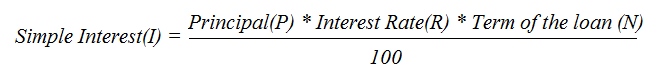Calculate Simple Interest using Principal Amount, Interest Rate, No. of Years | Simple Interest Calculator

# Calculate Simple Interest

## Calculate Simple Interest (I)

%

₹ 60,000
Simple Interest Amount
₹ 1,00,000
Principal Amount
₹ 1,60,000
Total Amount
37.50%
Interest % to Total Amount

## Simple Interest Calculator

#### What is Simple Interest ?

Simple interest is a method to calculate the interest rate on a loan payment. Interest is always depends on the original principle or sum, so interest on interest is not included.

#### What is Principal Amount ?

Principal is the sum of money on which the interest to be earned.

#### What is interest rate ?

Interest rate is the percentage at which interest accrued over time.

#### What is No. of Years ?

Time or period is the length of period in years.

## Formula to Calculate Simple Interest

The simple interest formula allows us to calculate I, which is the interest earned or charged on a loan. According to this formula, the amount of interest is given bywhere

• I is the interest amount,
• P is the principal amount,
• R is the annual interest rate in % form, and
• N is the loan period expressed in years.

## Simple Interest Example

John borrows 1,00,000 for 5 Years, at 10% simple interest rate.

##### Simple Interest Amount(I) = Principal(P) * Interest Rate(R) * Term of the loan (N) / 100
 Principal Amount(P) : 1,00,000 Interest Rate(R) : 10 % Term of the loan (N) : 5 Years

Simple Interest(I) = 1,00,000 * 0.10 * 5
Simple Interest(I) = 50,000